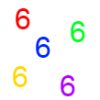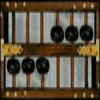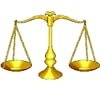Search by Topic

Resources tagged with Place value similar to Ruler:

Filter by: Content type:
Age range:
Challenge level:

There are 26 results

Broad Topics > Numbers and the Number System > Place valueClickety Click

Age 16 to 18 Short Challenge Level:

What is the sum of: 6 + 66 + 666 + 6666 ............+ 666666666...6 where there are n sixes in the last term?Sixty-seven Squared

Age 16 to 18 Challenge Level:

Evaluate these powers of 67. What do you notice? Can you convince someone what the answer would be to (a million sixes followed by a 7) squared?Binary Squares

Age 16 to 18 Challenge Level:

If a number N is expressed in binary by using only 'ones,' what can you say about its square (in binary)?Age 5 to 16

Nowadays the calculator is very familiar to many of us. What did people do to save time working out more difficult problems before the calculator existed?Age 16 to 18 Challenge Level:

If the last four digits of my phone number are placed in front of the remaining three you get one more than twice my number! What is it?Balance Power

Age 11 to 18 Challenge Level:

Using balancing scales what is the least number of weights needed to weigh all integer masses from 1 to 1000? Placing some of the weights in the same pan as the object how many are needed?What a Joke

Age 14 to 16 Challenge Level:

Each letter represents a different positive digit AHHAAH / JOKE = HA What are the values of each of the letters?Big Powers

Age 11 to 16 Challenge Level:

Three people chose this as a favourite problem. It is the sort of problem that needs thinking time - but once the connection is made it gives access to many similar ideas.Age 7 to 18

This article for the young and old talks about the origins of our number system and the important role zero has to play in it.Phew I'm Factored

Age 14 to 16 Challenge Level:

Explore the factors of the numbers which are written as 10101 in different number bases. Prove that the numbers 10201, 11011 and 10101 are composite in any base.Plus Minus

Age 14 to 16 Challenge Level:

Can you explain the surprising results Jo found when she calculated the difference between square numbers?Latin Numbers

Age 14 to 16 Challenge Level:

Can you create a Latin Square from multiples of a six digit number?Back to Basics

Age 14 to 16 Challenge Level:

Find b where 3723(base 10) = 123(base b).An Alphanumeric

Age 16 to 18

Freddie Manners, of Packwood Haugh School in Shropshire solved an alphanumeric without using the extra information supplied and this article explains his reasoning.Purr-fection

Age 16 to 18 Challenge Level:

What is the smallest perfect square that ends with the four digits 9009?DOTS Division

Age 14 to 16 Challenge Level:

Take any pair of two digit numbers x=ab and y=cd where, without loss of generality, ab > cd . Form two 4 digit numbers r=abcd and s=cdab and calculate: {r^2 - s^2} /{x^2 - y^2}.Number Rules - OK

Age 14 to 16 Challenge Level:

Can you convince me of each of the following: If a square number is multiplied by a square number the product is ALWAYS a square number...Composite Notions

Age 14 to 16 Challenge Level:

A composite number is one that is neither prime nor 1. Show that 10201 is composite in any base.Really Mr. Bond

Age 14 to 16 Challenge Level:

115^2 = (110 x 120) + 25, that is 13225 895^2 = (890 x 900) + 25, that is 801025 Can you explain what is happening and generalise?Novemberish

Age 14 to 16 Challenge Level:

a) A four digit number (in base 10) aabb is a perfect square. Discuss ways of systematically finding this number. (b) Prove that 11^{10}-1 is divisible by 100.Never Prime

Age 14 to 16 Challenge Level:

If a two digit number has its digits reversed and the smaller of the two numbers is subtracted from the larger, prove the difference can never be prime.Age 11 to 18

We are used to writing numbers in base ten, using 0, 1, 2, 3, 4, 5, 6, 7, 8, and 9. Eg. 75 means 7 tens and five units. This article explains how numbers can be written in any number base.Enriching Experience

Age 14 to 16 Challenge Level:

Find the five distinct digits N, R, I, C and H in the following nomogram2-digit Square

Age 14 to 16 Challenge Level:

A 2-Digit number is squared. When this 2-digit number is reversed and squared, the difference between the squares is also a square. What is the 2-digit number?Basic Rhythms

Age 16 to 18 Challenge Level:

Explore a number pattern which has the same symmetries in different bases.Multiplication Magic

Age 14 to 16 Challenge Level:

Given any 3 digit number you can use the given digits and name another number which is divisible by 37 (e.g. given 628 you say 628371 is divisible by 37 because you know that 6+3 = 2+7 = 8+1 = 9). . . .